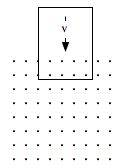# Loop falling into a constant magnetic field

## Homework Statement(Not this exactly, but it gets the idea across)

I have a square loop of wire with sides L and at t=0, I have the bottom side of the loop right above a uniform magnetic field. So the flux is 0 at t=0, and right when I drop the loop, it is forced downwards at a magnitude of mg. My question is, for the time the flux is changing there is a current induced, but also for that time, the top side is not in the magnetic field.

So do I also have to treat the bottom side as a straight wire that is being accelerated in a magnetic field? Therefore there will be an upwards force that counteracts the mg downwards. If so, how would I calculate that upward force because the current in the wire is being induced by the magnetic field, so it seems F=ILB wouldn't work for this.

## Homework Equations

F=ILB
a=mg-(upwards force)
emf=-dΦ/dt

## The Attempt at a Solution

I just have a fundamental question about the problem, so there's not really an attempt...